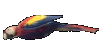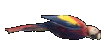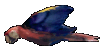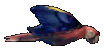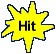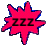Math Help - Subsets & Sets - Game Tips:

- In this game, the rectangular shape 'U' represents the Universal Set of members for all possible sets.

- If set P is a circular region inside U, then the set 'not P' is the region outside circle P and inside U.
The set 'not P' refers to all members of set U that are not members of set P.

- When selecting members from two sets, the word 'and' refers to the overlapping region P and Q.
P and Q is called the intersection set.
If set P is completely inside set Q, then P is called a subset of Q resulting in (P and Q) = P.
If set Q is completely inside set P, then Q is called a subset of P resulting in (P and Q) = Q.
If set P and set Q do not overlap ,
then they are disjoint sets with the result (P and Q) = empty = Null set.

- When selecting members from two sets, the word 'or' refers to the entire region including P or Q.
P or Q is called the union set.    [The 'exclusive or' concept is not used in this game.]
If set P is completely inside set Q, then P is a subset of Q resulting in (P or Q) = Q.
If set Q is completely inside set P, then Q is a subset of P resulting in (P or Q) = P.

- Your Game Score is reduced by the number of parrot hits.

- To slow the game speed repeat tap/click on the word Slider.
- To increase the game speed repeat tap/click on the word Math.
- Speed can also be adjusted with a keyboard's - and + keys.

- Refresh/Reload the web page to restart the game.